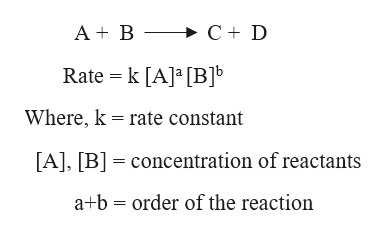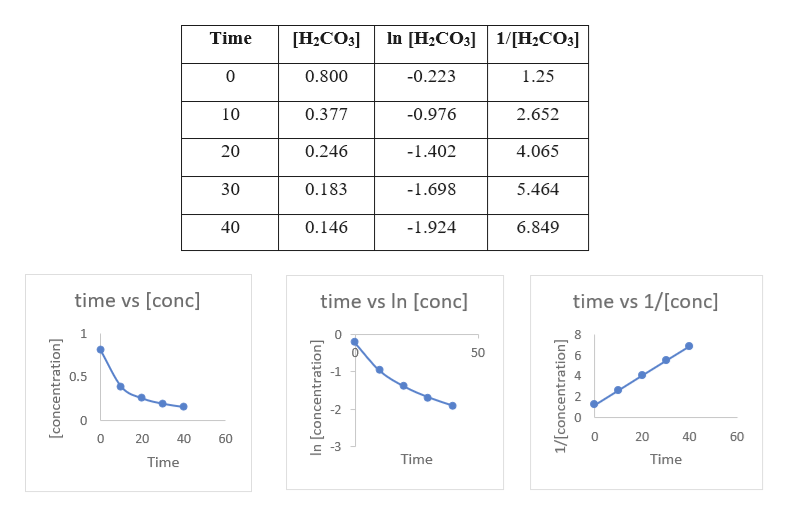DarianaDeducing a rate law from the change in concentration over timeA chemistry graduate student is studying the rate of this reaction:H2CO, (ag) H20 (ag)+Co, (ag)She fills a reaction vessel with HCO2 and measures its concentration as the reaction proceeds:H,Co3]timeminutes)00.800 M10.0.377 M20.0.246 M300.183 M40.0.146 MUse this data to answer the following questions.rate kWrite the rate law for this reaction.Calculate the value of the rate constant k.?XRound your answer to 2 significant digits. Also besure your answer has the correct unit symbol. DarianaUsing an integrated rate law for a first-order reactionConsider this reaction2HI (g)H (g)+I, (e)At a certain temperature it obeys this rate law.rate (0.00981 s[HI]Suppose a vessel contains HI at a concentration of 0.460 M. Calculate the concentration of HI in the vessel 65.0 seconds later. You may assume no otherreaction is important.Round your answer to 2 significant digits?X

Question
Step 1

Since there are multiple questions, we will answer only the first one. If you require the answer of the second question, please repost it separately. Thank you.

Step 2

The rate law of a reaction is expressed as:help_outlineImage TranscriptioncloseA B >C+ D Rate k [A] [BJb Where, k rate constant [A], [B concentration of reactants a+b order of the reaction fullscreen
Step 3

Now, we need to find the order of the reaction. This can be done by plotting graphs of time versus concentration. If the time vs [conc] graph gives a straight line, it will be a zero order re...help_outlineImage TranscriptioncloseIn [H2CO3 Time [H2CO3] 1/H2CO3] 0 0.800 -0.223 1.25 0.377 2.652 10 -0.976 20 0.246 -1.402 4.065 30 5.464 0.183 -1.698 40 0.146 -1.924 6.849 time vs [conc] time vs In [conc] time vs 1/[conc] .NK. 50 0.5 0 20 40 60 0 20 40 60 Time Time Time fullscreen

Want to see the full answer?

See Solution

Want to see this answer and more?

Our solutions are written by experts, many with advanced degrees, and available 24/7

See Solution
Tagged in

Chemical Kinetics